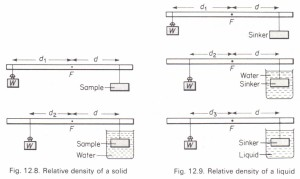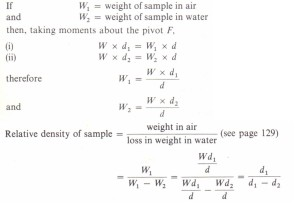# To measure the relative density of solids and liquids by a balanced lever

To measure the relative density of solids and liquids by a balanced lever

Relative density of a solid
A sample of the test solid is attached by a loop of thread to a metre rule pivoted near its mid-point and is balanced by the weight W, of any suitable mass, hung on the opposite side (Fig. 12.8).To measure the relative density of solids and liquids by a balanced lever

The distance d of the sample from the pivot is kept constant and the distance of W from the pivot is noted (d[). The sample is then immersed in water in a beaker and the balance restored by moving W closer to the pivot (d2).

Several pairs of values of d1 and d2 may be obtained from which a mean value for the relative density may be calculated. One advantage of this method is that no masses or weights have to be measured.

Relative density of a liquid
Two experiments are carried out exactly as described above in which the sample is replaced by any convenient sinker (e.g., a metal cylinder). These are followed by a third experiment with the sinker immersed in the liquid whose relative density is required (Fig. 12.9). By reasoning similar to the above it may be shown that, loss in weight of sinker in liquid relative density of liquid = . . f . k. (see page 130) loss III weight 0 Sill er III water.The steps used in arriving at this formula should be shown III full when the experiment is written up in the practical notebook.To measure the relative density of solids and liquids by a balanced lever
Share This